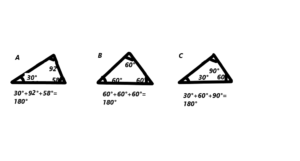# Math in Focus Grade 7 Chapter 7 Lesson 7.3 Answer Key Constructing Triangles

Practice the problems of Math in Focus Grade 7 Workbook Answer Key Chapter 7 Lesson 7.3 Constructing Triangles to score better marks in the exam.

## Math in Focus Grade 7 Course 2 B Chapter 7 Lesson 7.3 Answer Key Constructing Triangles

### Math in Focus Grade 7 Chapter 7 Lesson 7.3 Guided Practice Answer Key

Construct the triangle from the given information. Use a compass and ruler.

Question 1.
Triangle PQR: PQ = 5.6 cm, QR = 4.5 cm, and PR = 8.2 cm.

The measurements of the triangle are PQ=5.6cm ,PR=8.2cm and QR=4.5cm.

Eplanation: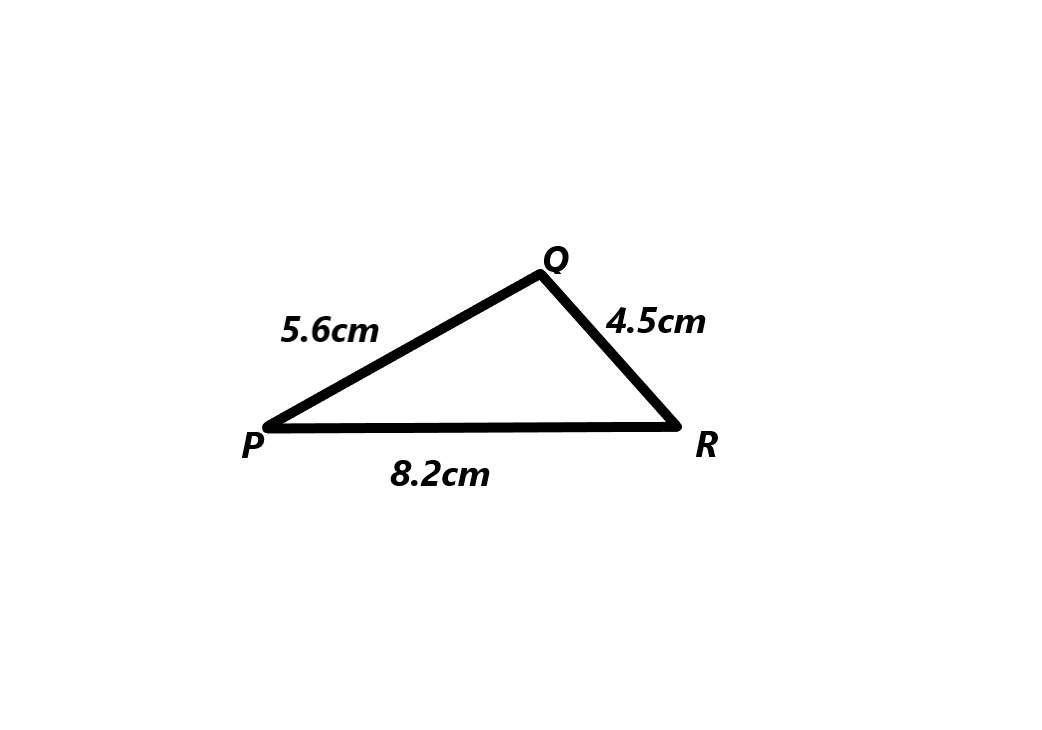Construct the triangle from the given information. Use a ruler and compass.

Question 2.
Triangle ABC: BC = 4 cm, m∠ABC = 25°, and m∠ACB = 120°.
The measurements of the triangle are PQ=5.6cm ,PR=8.2cm and QR=4.5cm.

Explanation: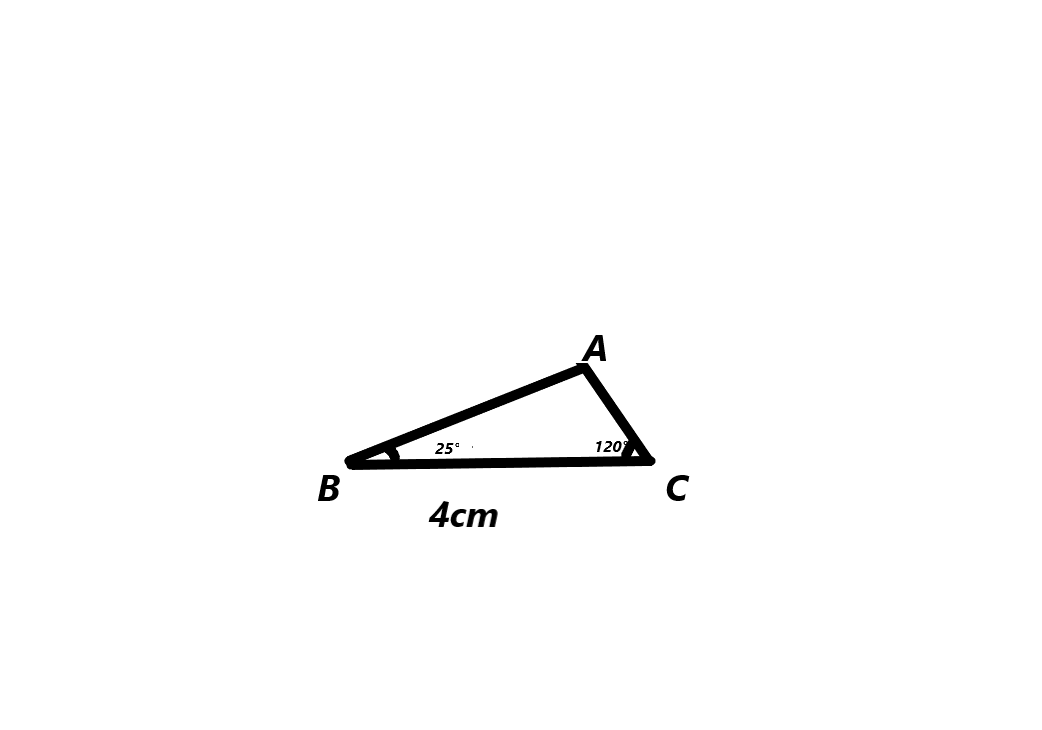Question 3.
Triangle KLM: KL = 8.2 cm, KM = 6.9 cm, and m∠LKM = 75°.
The measurements of the triangle KLM are KL=8.2cm ,KM=6.9cm and the angle LKM=75°.

Explanation: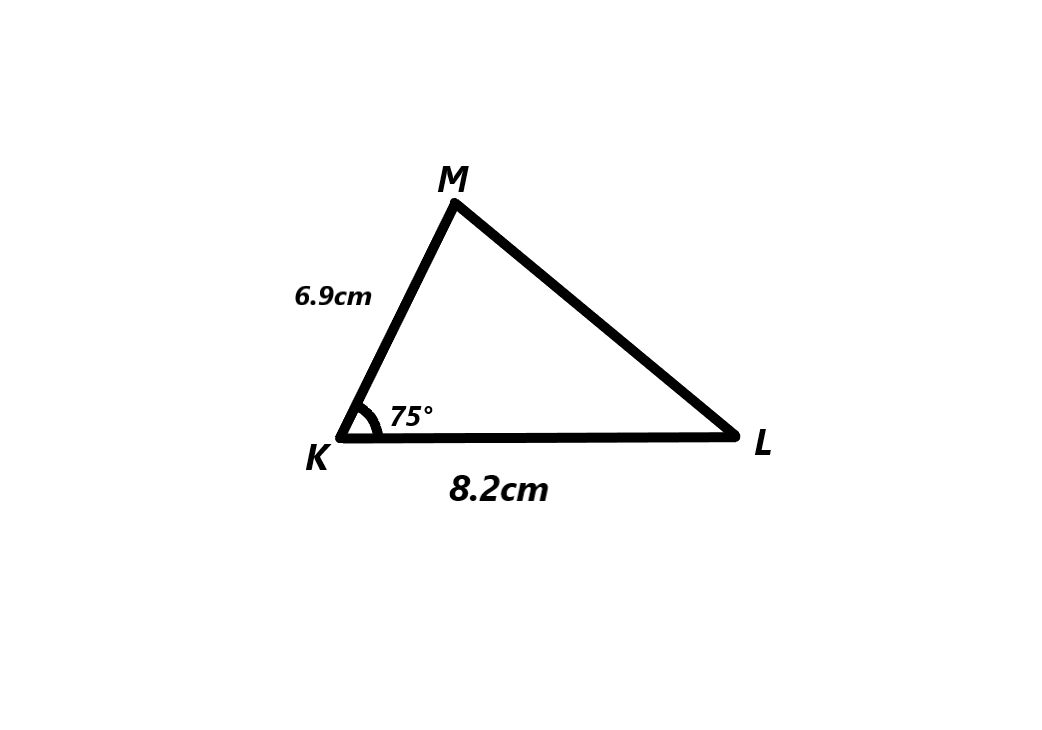Construct the triangle from the given information. Use a compass, ruler, and protractor.

Question 4.
Triangle KLM: KL = 7cm, KM = 9 cm, and m∠KLM = 125°.
The measurements of the triangle KLM are KL=7cm , KM=9cm and m∠KLM= 125°.

Explanation: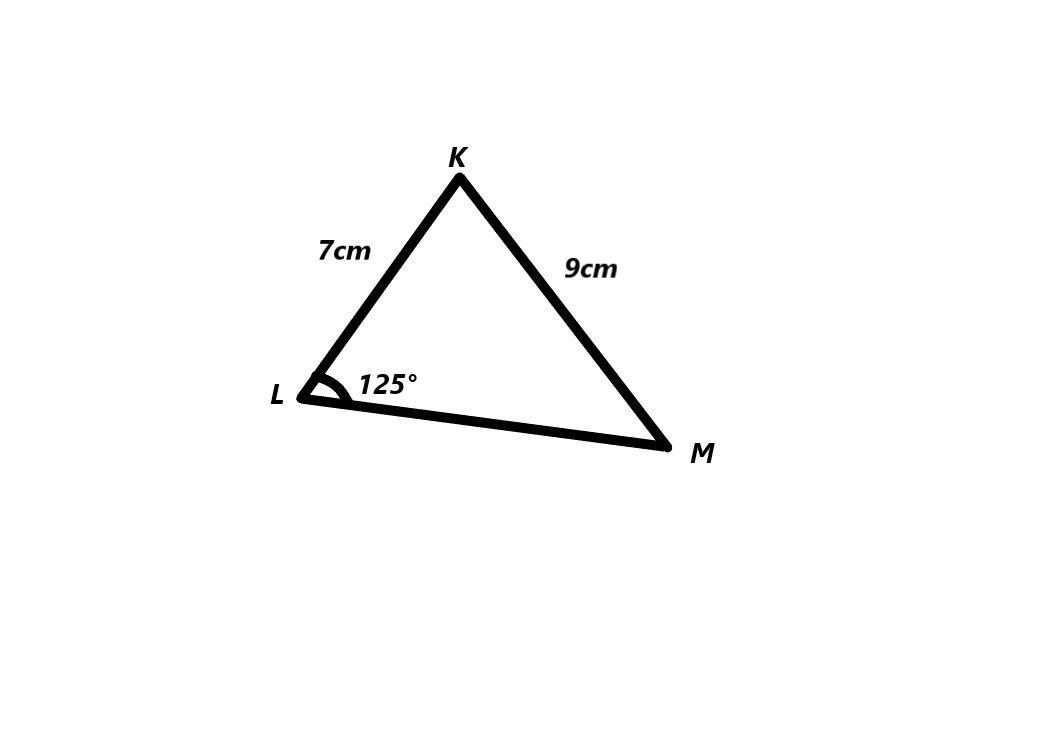Hands-On Activity

Materials

• protractor
• compass
• ruler

Decide Whether Given Measures Can Be Used To Construct One Triangle, More Than One Triangle. Or No Triangles

Work in pairs.

Step 1.
Try to construct triangle ABC with AB = 7 cm, BC = 8 cm, and DE = 4.6 cm, EF = 6 cm, and AC = 11 cm.
The measurements of the triangle ABC are  AB = 7 cm, BC = 8 cm, and DE = 4.6 cm, EF = 6 cm, and AC = 11 cm.

Explanation: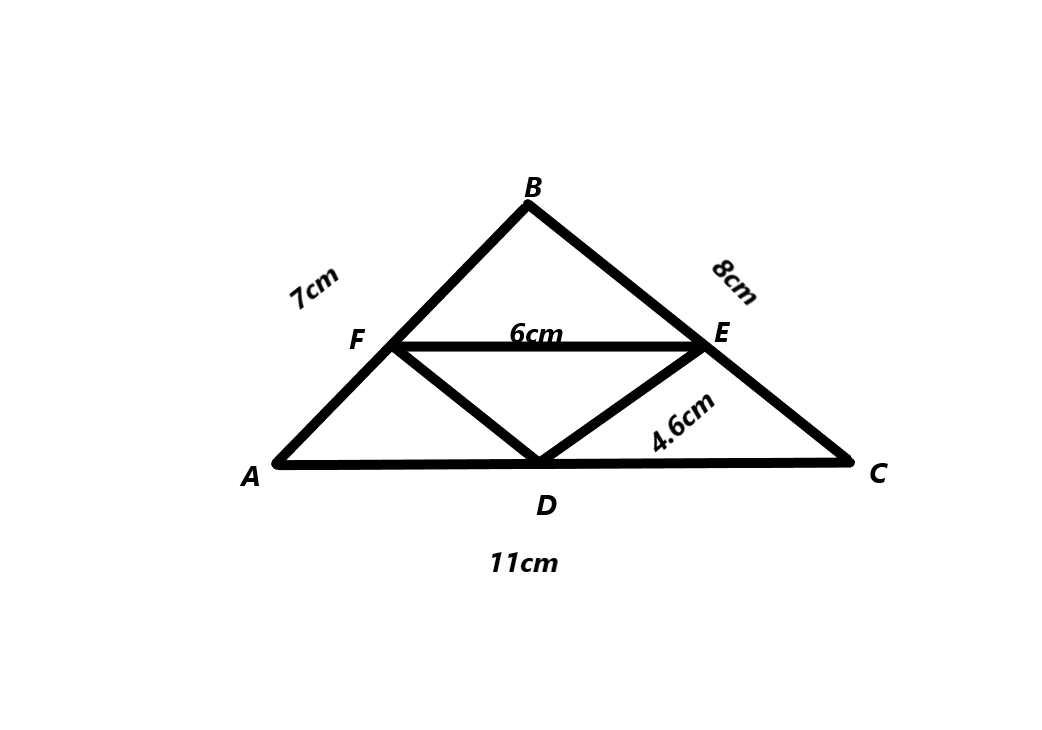Step 2.
Try to construct triangle DEF with DE = 4.6 cm, EF = 6 cm, and DF = 12 cm.
The measurements of the triangle DEF are DE = 4.6 cm, EF = 6 cm, and DF = 12 cm.

Explanation: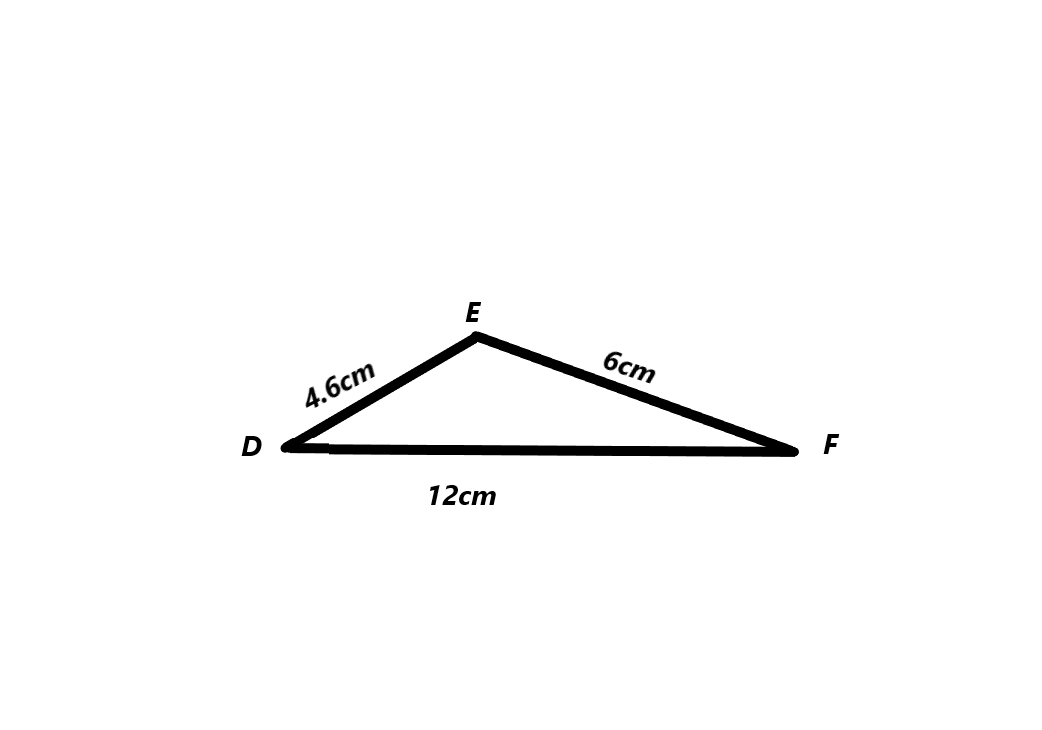Step 3.
Try to construct triangle GHI with GH = 6 cm, HI = 5 cm, and JK = 6 cm, JL = 4.7 cm, and m∠GHI = 50°.

Step 4.
Try to construct triangle JKL with JK = 6 cm, JL = 4.7 cm, and m∠JKL = 50°.
The measurements of the triangle JKL are JK = 6 cm, JL = 4.7 cm, and m∠JKL = 50°.

Explanation: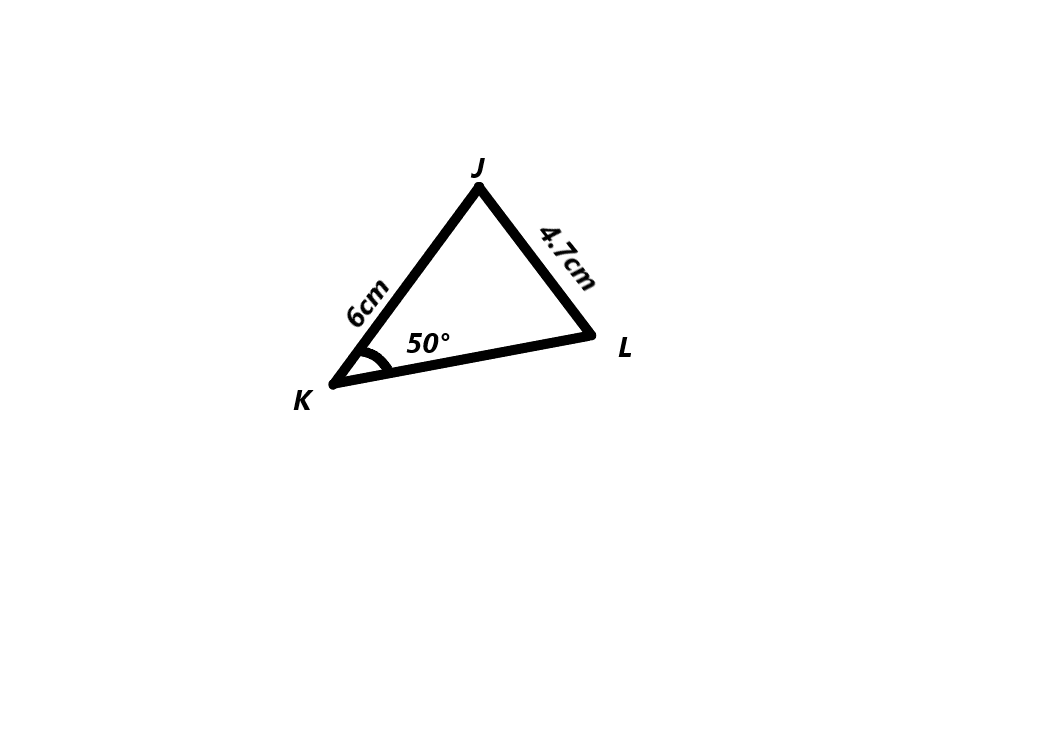Step 5.
Try to construct triangle MNP with MN = 7 cm, m∠MNP = 60°, and m∠PMN = 40°.
The measurements of the triangle MNP are MN = 7 cm, m∠MNP = 60°, and m∠PMN = 40°.

Explanation: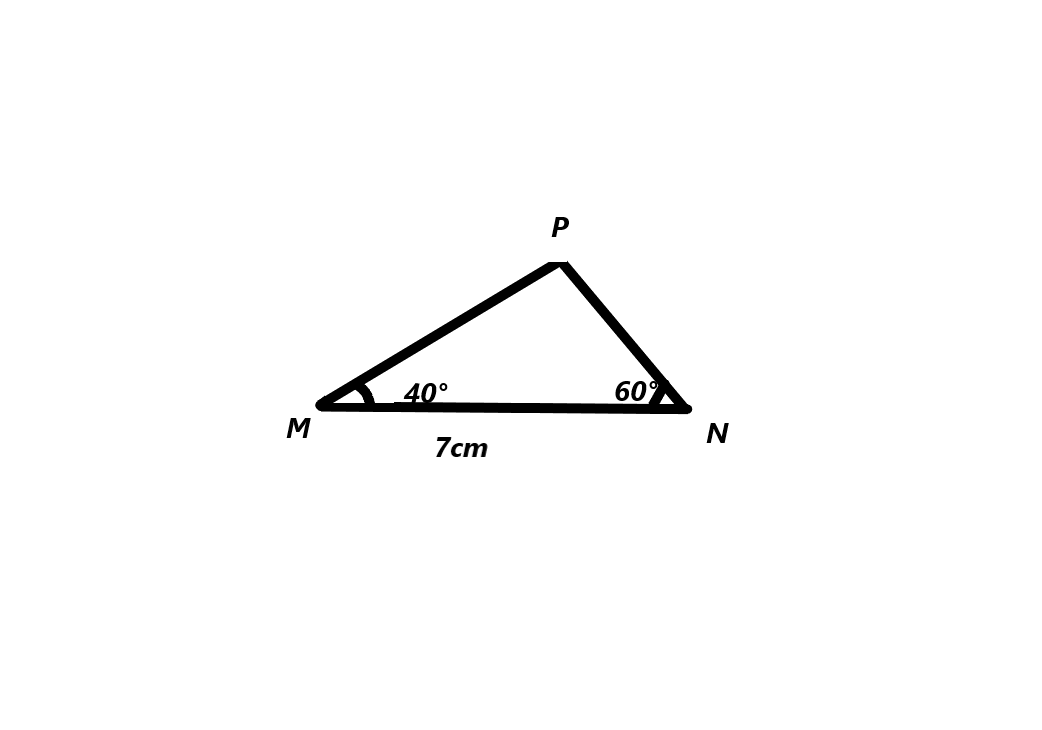Step 6.
Were there any triangles you could not construct? Were there any triangles that you could construct in more than one way? Explain.
Yes, at step 3 we could not construct any triangle. At step 1 we can construct more than one triangle because the measurements of the triangle ABC are  AB = 7 cm, BC = 8 cm, and DE = 4.6 cm, EF = 6 cm, and AC = 11 cm.

Math Journal
Use your results to decide whether you can always construct exactly one triangle from the given information. Justify your answer.

a) Given three side lengths

b) Given two side lengths and an angle measure

Find the number of triangles that can be constructed. Try constructing the triangles to make your decision.

Question 5.
PQ = 4.8 cm, QR = 5.4 cm, and m∠PQR = 100°.
The measurements of the triangle PQR = PQ = 4.8 cm, QR = 5.4 cm, and m∠PQR = 100°.

Explanation: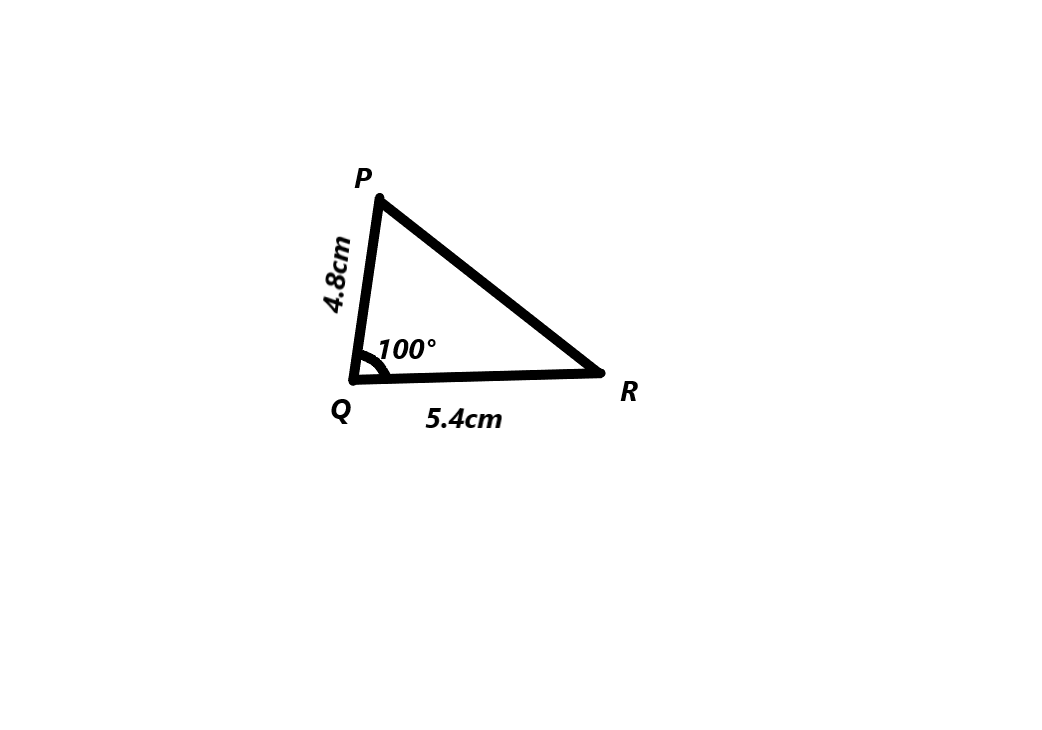Question 6.
AB = 6.2 cm, BC = 4.8 cm, and m∠BAC = 75°.
The measurements of the triangle ABC are AB = 6.2 cm, BC = 4.8 cm, and m∠BAC = 75°.

Explanation: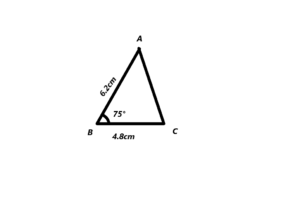Question 7.
ST = 7.7 cm, SU = 5.2 cm, and m∠STU = 40°.
The measurements of the triangle STU are ST = 7.7 cm, SU = 5.2 cm, and m∠STU = 40°.

Explanation: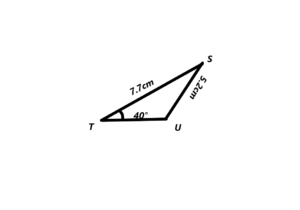### Math in Focus Course 2B Practice 7.3 Answer Key

Use the given information to construct each triangle.

Question 1.
In triangle CDE, CD = 7 cm, DE = 4 cm, and CE = 6.5 cm.
The measurements of the triangle CDE , CD = 7 cm, DE = 4 cm, and CE = 6.5 cm.

Explanation: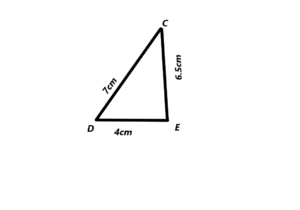Question 2.
In triangle ABC, BC = 6 cm, m∠ABC = 30°, and m∠ACB = 60°. Find m∠BAC and AC.
The measurements of the triangle ABC , BC = 6 cm, m∠ABC = 30°, and m∠ACB = 60°.
B= 30° and C= 60°
A=180°-B-C
A=180°- 30°- 60°
A=90°.
AC=3cm.

Explanation: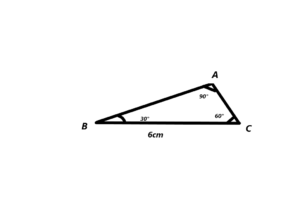Question 3.
In an equilateral triangle, each side length is 6.5 centimeters long.
The measurements of the equilateral triangle , each side length is 6.5 cm.

Explanation: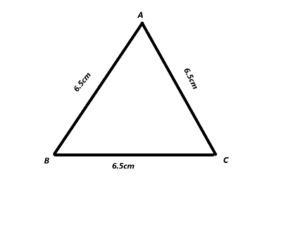Question 4.
In triangle ABC, AB = 4 cm, AC = 5 cm, and m∠ABC = 40°.
The measurements of the triangle ABC, AB = 4 cm, AC = 5 cm, and m∠ABC = 40°.

Explanation: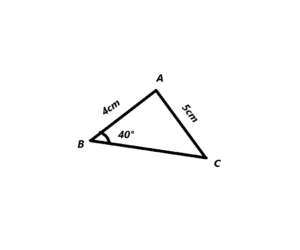Question 5.
In triangle ABC, AB = 6 cm, BC = 8 cm, and AC = 10 cm. What kind of triangle is triangle ABC? Classify it by both sides and angles.
The measurements of the triangle ABC, AB = 6 cm, BC = 8 cm, and AC = 10 cm. The triangle ABC is a right angle triangle. Right angle triangle has 3 sides and it have one angle  90°.

Explanation: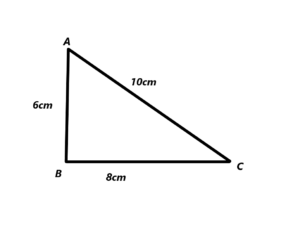Question 6.
In triangle XYZ, XV = XZ = 4 cm and YZ = 5 cm. Find m∠XZY.
The measurements of the triangle XYZ, XV = XZ = 4 cm and YZ = 5 cm.
The angle of the XZY is 50°.

Explanation: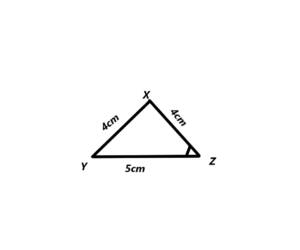Question 7.
Triangle POR has the dimensions shown in the diagram.

a) Construct triangle POR.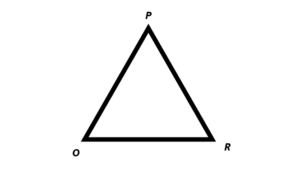b) Using a ruler, from your construction, measure the length of $$\overline{P R}$$.
Using a ruler we measure the length of $$\overline{P R}$$.
PR ≈ 6.1 cm

c) Find the measures of ∠P and ∠R without using a protractor. Justify your answer.
△PQR is Isosceles because PQ = QR Therefore the angles P and R we congruent:
m∠P = m∠R
Find m∠P and m∠R:
m∠P + m∠R – m∠Q = 180°
2m∠P + 100° = 180°
2m∠P = 180° – 100°
2m∠P = 80°
m∠P = m∠R = $$\frac{80^{\circ}}{2}$$ = 40°

Question 8.
Math Journal
Is it possible to construct a triangle PQR in which PQ = 12 cm, PR = 5 cm, and QR = 4cm? Explain.
We cannot construct a triangle PQR in which PQ = 12 cm, PR = 5 cm, and QR = 4cm because the lengths of the PR , QR are very small.

Explanation: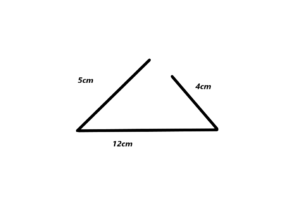Question 9.
Three triangles have angle measures of 500 and 600. In one triangle, the side included between these angles is 2 centimeters. In the second triangle, the included side length is 3 centimeters, and in the third triangle, the included side length is 4 centimeters.

a) Construct the three triangles.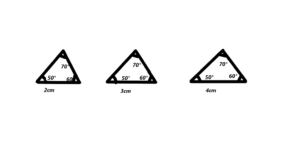b) In each triangle, what is the measure of the third angle?
Sum of angles of triangle is 180 so the given triangles have angle measures of 50 degree and 60 degree another angle is 70 degree.

c) Using the triangles constructed to help you, what can you deduce about the number of triangles that can be constructed if you are given three angle measures of a triangle but not the measure of any side length?